Definition: circumference from Philip's Encyclopedia

Distance round the boundary of a plane geometric figure, nearly always applied to a circle, for which it has the value 2πr, where r is the radius.

Summary Article: Circumference of a circle (16.2)
From Teach Yourself: Mathematics: A Complete Introduction

Circumference is the special name of the perimeter of a circle, that is, the distance all around it. The word is derived from the Latin circum, meaning ‘round’, and fero, meaning ‘carry’.

If you measure the circumferences and diameters of some circular objects and then, for each one, work out the value of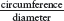, you should find that your answer is always just over 3. In fact, the value of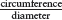is the same for every circle and is denoted by the Greek letter π, pronounced ‘pie’. So for every circle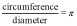William Jones, an Englishman, was, in 1706, the first mathematician to use π in this way, probably because π is the first letter of the Greek word peripheria, meaning ‘periphery’ or ‘boundary’.

Archimedes (c. 225 bc) showed that the value of π is between and . Using modern computing techniques, its value has been calculated to over six billion decimal places but it cannot be found exactly as the decimal never recurs or terminates.

To perform calculations involving π, use the π key on your calcu­lator. If you do not have a π key, 3.14 or 3.142 is sufficiently accurate for all practical purposes.

Using C to stand for the circumference of a circle and d to stand for its diameter, you can write the result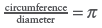. as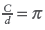. Multiplying both sides by d, you obtain the formula C = pd. So, to find the circumference of a circle, you multiply its diameter by π. The same units must be used for the circumference and the diameter.

If you are told the radius of a circle and want to find its circumference, you could first double the radius to obtain the diameter and then multiply the result by π.

Alternatively, using r to stand for the radius of the circle, you can replace d by 2r in the formula C = pd. This gives C = π × 2r, usually written as C = 2 p r. In this formula, the same units must be used for the circumference and the radius.

If you know the circumference of a circle and want to find its diameter, you could substitute the value of C in the formula C = πd and then solve the resulting equation.

Alternatively, you can make dthe subject of the formula C = pd.

Dividing both sides by π gives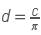. So, to find the diameter of a circle, you divide its circumference by π.

If you know the circumference of a circle and want to find its radius, you could find the diameter of the circle and then halve your answer.

Alternatively, you could make r the subject of the formula C = 2π.

Dividing both sides of the formula by 2π gives . So, to find the radius of a circle, you divide its circumference by 2π.© Hodder Education, an Hachette UK Company 2013

### Related Articles

The Hutchinson Unabridged Encyclopedia with Atlas and Weather Guide

Straight line from the centre of a circle to its circumference, or from the centre to the surface of a sphere. essays Circles: working out area, diam

##### Full text Article simple harmonic motion
The Macquarie Dictionary

1. a form of vibratory motion which may be represented by projecting on to the diameter of a circle the uniform motion of a point round its circu